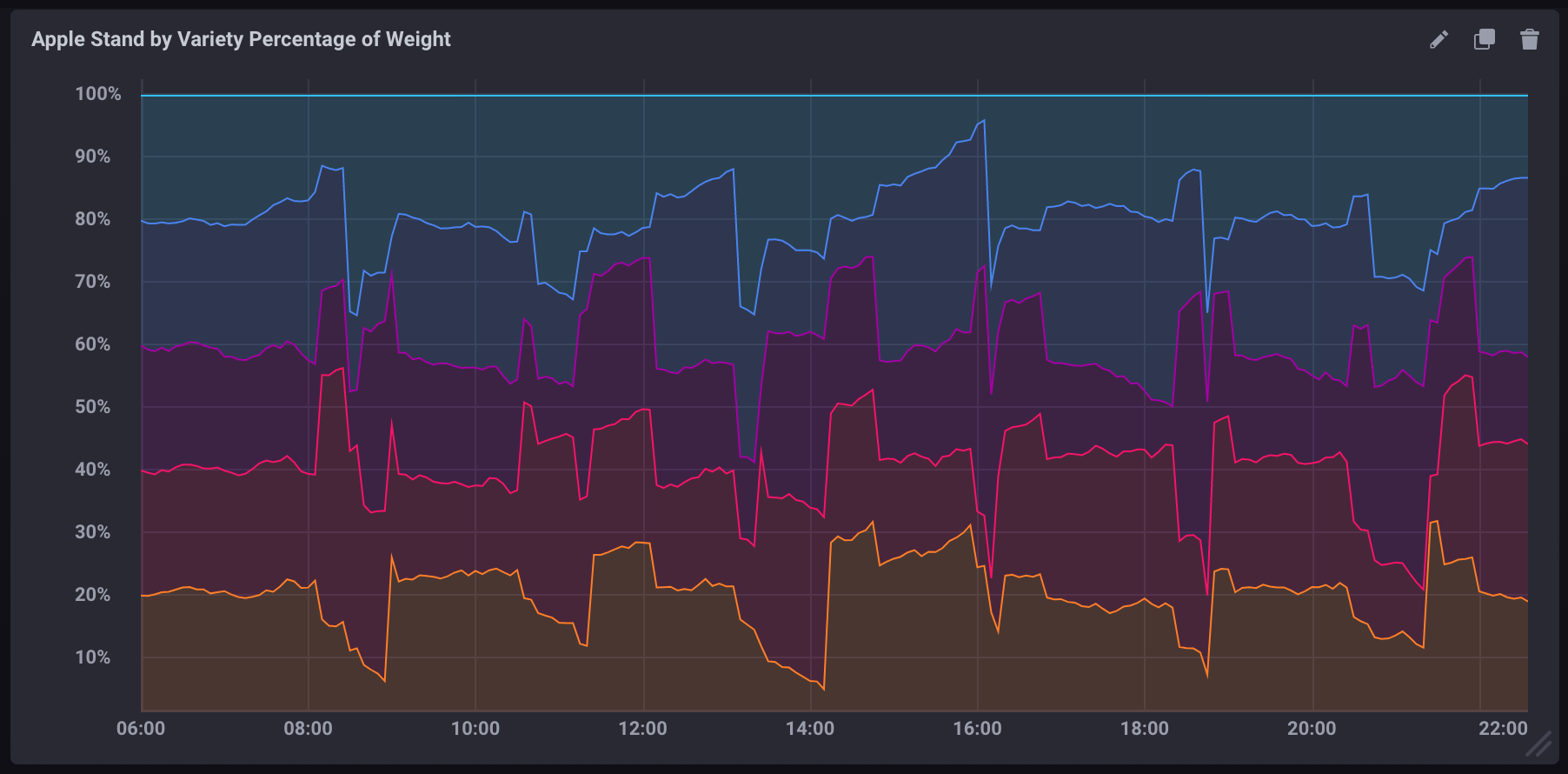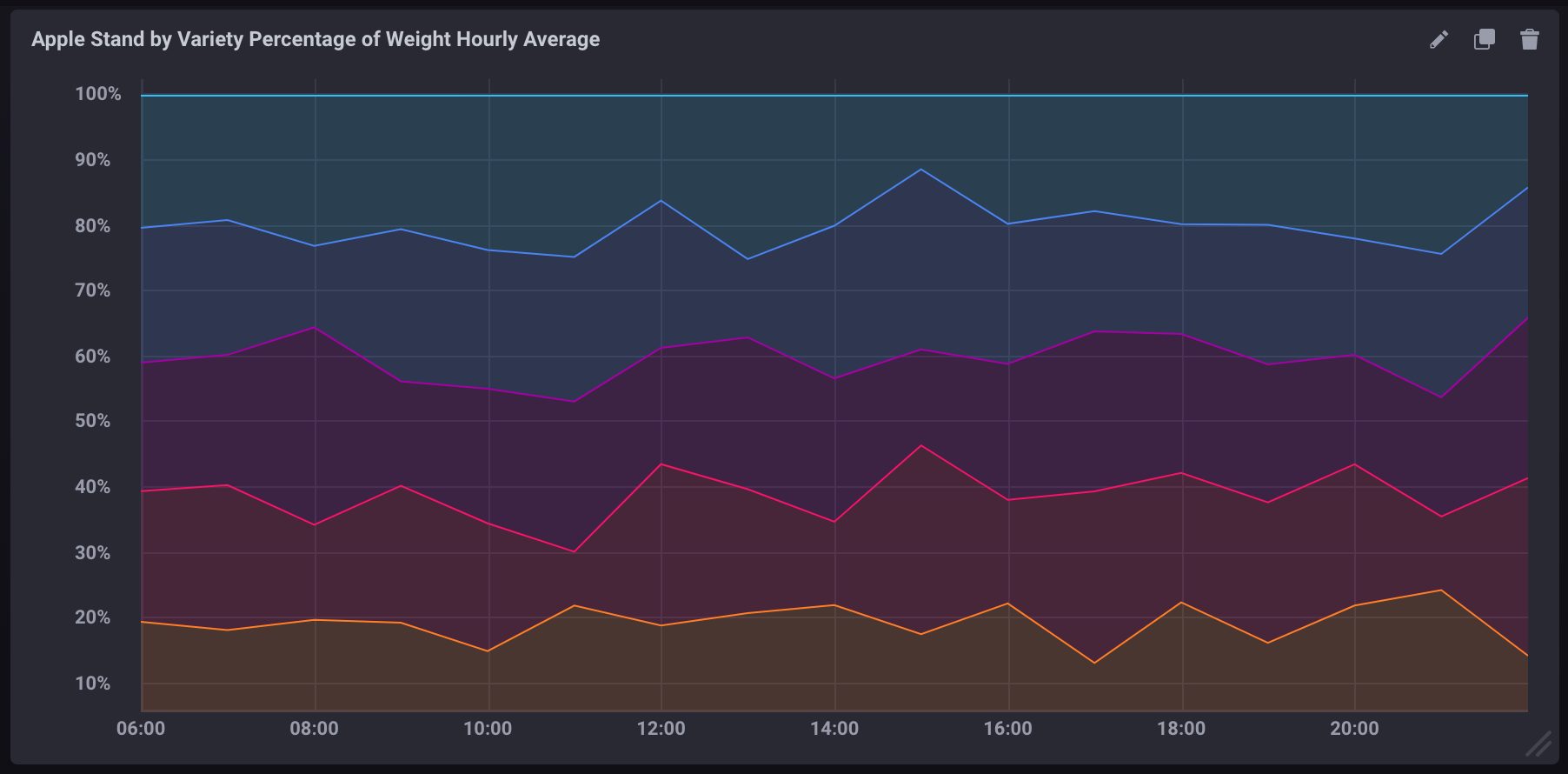Search
Document Center

# Calculate percentage in queries

Last Updated: May 29, 2020

InfluxQL allows you to solve simple math equations. This simplifies the calculation of percentages based on two fields in a measurement. Read the following notes before you calculate percentages.

# Basic calculations in a query

`SELECT` statements support the use of basic math operators, such as `+`, `-`, `/`, `*`, and `()`.

``-- Add two field keys``SELECT field_key1 + field_key2 AS "field_key_sum" FROM "measurement_name" WHERE time < now() - 15m``-- Subtract one field key from another``SELECT field_key1 - field_key2 AS "field_key_difference" FROM "measurement_name" WHERE time < now() - 15m``-- Group field keys for calculation and chain the calculation results``SELECT (field_key1 + field_key2) - (field_key3 + field_key4) AS "some_calculation" FROM "measurement_name" WHERE time < now() - 15m``

# Calculate percentages in a query

You can calculate a percentage by dividing one field value by another and multiplying the result by 100.

``SELECT (field_key1 / field_key2) * 100 AS "calculated_percentage" FROM "measurement_name" WHERE time < now() - 15m``

# Calculate a percentage by using aggregate functions

If you use aggregate functions to calculate a percentage, all data in the query must be referenced by using aggregate functions. You cannot mix aggregated data and non-aggregated data.

All aggregate functions must include a `GROUP BY time()` clause to define the time interval at which points are grouped and aggregated.

``SELECT (sum(field_key1) / sum(field_key2)) * 100 AS "calculated_percentage" FROM "measurement_name" WHERE time < now() - 15m GROUP BY time(1m)``

# Examples

## Sample data

The following example uses simulated Apple Stand data that tracks the weight of baskets that contain different varieties of apples throughout the day.
2. Import the sample data.

``influx -ssl -username <Username> -password <Password> -host <Domain name> -port 3242 -import -path=path/to/apple_stand.txt -database=apple_stand``

## Calculate the percentage of total weight per apple variety

The following query calculates the percentage of the total weight each apple variety accounts for at each specified time point.

``SELECT``    ("braeburn"/total_weight)*100,``    ("granny_smith"/total_weight)*100,``    ("golden_delicious"/total_weight)*100,``    ("fuji"/total_weight)*100,``    ("gala"/total_weight)*100``FROM "apple_stand"."autogen"."variety"``

In the following figure, the calculation result is visualized as a stacked graph in Chronograf.## Calculate the aggregate percentage per apple variety

The following query calculates the percentage of the average weight of each apple variety in the average weight of all apples in each hour.

``SELECT``    (mean("braeburn")/mean(total_weight))*100,``    (mean("granny_smith")/mean(total_weight))*100,``    (mean("golden_delicious")/mean(total_weight))*100,``    (mean("fuji")/mean(total_weight))*100,``    (mean("gala")/mean(total_weight))*100``FROM "apple_stand"."autogen"."variety"``WHERE time >= '2018-06-18T12:00:00Z' AND time <= '2018-06-19T04:35:00Z'``GROUP BY time(1h)``

Note the following items about the query:

• This query uses aggregate functions (`mean()`) to extract all data.
• This query includes a `GROUP BY time()` clause that aggregates data into 1 hour blocks.
• This query is performed based on a specified time window. If this time window is not specified, the aggregate functions may consume a large number of compute resources.

In the following figure, the query result is visualized as a stacked graph in Chronograf.InfluxDB® is a trademark registered by InfluxData, which is not affiliated with, and does not endorse, TSDB for InfluxDB®.